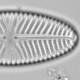## Testing the significance of Random Forest reconstructions

Telford and Birks (2011) introduce a method for testing the statistical significance of a palaeoenvironmental reconstruction from biotic assemblages. The palaeoSig package (Telford, 2012) for R will run the significance tests using any of the reconstruction methods in the rioja package (Juggins, 2012). This includes most of the commonly used methods, including weighted averaging (WA()), weighted averaging partial least squares (WAPLS()), and the modern analogue technique (MAT()). The fun argument in randomTF() specifies which transfer function method should be used. The palaeoSig package will not, by default, work with other implementations of the commonly used methods, such as in the analogue package (Simpson, 2012), or machine learning methods such as neural networks and regression trees, as their arguments are different, and the predictions have a different format to those from rioja.

Fortunately, it is easy to write wrapper functions that pre- and post-process the data so that the statistical significance of a reconstruction based on any transfer function method can be used with randomTF(). I’m going to demonstrate this by testing the statistical significance of a pH-reconstruction for the Round Loch of Glenhead estimated with a random forest.

Random forests, implemented in the randomForest package, are a tree-based regression or classification method. They are simple to fit, with nothing like the complexity of a neural network, fairly fast to run, and have some useful diagnostic features.

We can start by loading the packages and data, and fitting a randomForest() model and making predictions. One complication is that we need to deal with taxa present only in the calibration set or the fossil data. With rioja, this is dealt with for us. Here we can create consistent species lists using the Merge() function. randomForest() uses a formula notation, as we are using this in an inverse sense, the environmental variable pH is the response, and the species data are the predictors. I’m using the “~.” notation, which means use all predictors, rather than typing them out “~spp1+spp2+…+sppn”, which is tedious and error prone.

```library(randomForest)
library(palaeoSig)

data(SWAP)
data(RLGH)

all.spp<-Merge(SWAP\$spec, RLGH\$spec, split=T)

#fit randomForest
rfmod<-randomForest(pH~., data=cbind(pH=SWAP\$pH,all.spp\$SWAP))
rfmod
sqrt(rfmod\$mse[length(rfmod\$mse)])#RMSEP
pred<-predict(rfmod, newdata=all.spp\$RLGH)

#WA for comparison
WAmod<-WA(sqrt(SWAP\$spec), SWAP\$pH)
performance(crossval(WAmod, method="boot", nboot=1000))
WApred<-predict(WAmod, sqrt(RLGH\$spec))

x11()
plot(RLGH\$depth\$Age, pred, col=2, type="b", pch=20, xlab="Age yr", ylab="pH")
lines(RLGH\$depth\$Age, WApred\$fit[,1], col=4, type="b", pch=20)#compare with WA
legend("topleft", legend=c("random forest", "weighted average"), pch=20, col=c(2,4), lty=1, bty="n")

```

The performance of the random forest model is slightly better than that of the weighted average model with inverse deshrinking (RMSEP 0.317 vs 0.323; r2 0.831 vs 0.825), and the reconstructions share the same trend, but differ in detail.pH reconstruction for the Round Loch of Glenhead using random forests (red) or weighted averaging (blue).

To test the statistical significance of this random forest reconstruction, we need to make a wrapper function that takes the data from randomTF() that was prepared for rioja and formats it in the way that randomForest() expects. We then need to make a new predict function that will return the data in the same format as a rioja function would.

```rfWrap<-function(spp, ev,...){
mod<-randomForest(ev~. ,data=cbind(ev=ev,spp))
res<-list(mod=mod)
class(res)<-"rfWrap"
res
}

predict.rfWrap<-function(object,newdata,...){
pred<-predict(object\$mod, newdata=newdata)
list(fit=data.frame(p=pred))
}

rTF<-randomTF(sqrt(all.spp\$SWAP), data.frame(pH=SWAP\$pH), fos=sqrt(all.spp\$RLGH), fun=rfWrap, col=1, n=99)
rTF
plot(rTF)
```

The main purpose of rfWrap() is to change the data from a species data.frame and an environmental vector into a formula notation with data argument and to pass this to randomForest(). The model output is put into the list res, which could also contain diagnostics needed to select the best model. The class of res is set to rfWrap so that the new predict function will be used. predict() is a generic function; an appropriate function for the class of object will be invoked. If the object is of class “xxx”, predict.xxx() will be called. rfWrap() returns an object with class rfWrap, so predict() will call predict.rfWrap() to make the predictions. All predict.rfWrap() does is send the model (class randomForest) and newdata back to predict, which will in turn call predict.randomForest(). predict.rfWrap() puts the predictions into a data.frame and puts this into a list with the name fit. This mimics the format of predictions from functions in the rioja package. Future versions of randomTF() might relax these requirements if I can find a robust way to do it.

In this case the reconstruction in statistically significant (p=0.01). Note that tree-based methods are unaffected by monotonic transformations of the predictors, but the ordination used in randomTF() is affected, and transforming the species data is usually a good idea. With untransformed data, most of the weight is on the few most dominant species. Currently the ordination and the reconstruction automatically use the same data transformation. This could be modified in a future version if it is a problem.Histogram showing the proportion of the variance in the Round Loch of Glenhead diatom record explained by reconstructions of 99 random environmental variables and the pH reconstruction (black line). The dashed red line represents the 95th percentile of the null distribution. The dashed black line marks the amount of variance explained by the first axis of the RDA.

Machine learning techniques, generally take much longer than weighted averaging, and it may not be feasible to run a reasonable number of trials for the null model. There may be ways to optimise some of the process, for example if reconstructions from multiple lakes are being tested, the transfer function models for the simulated environmental variables only need to be fitted once, and then predictions made for each lake. This might be implemented in the next version of palaeoSig.